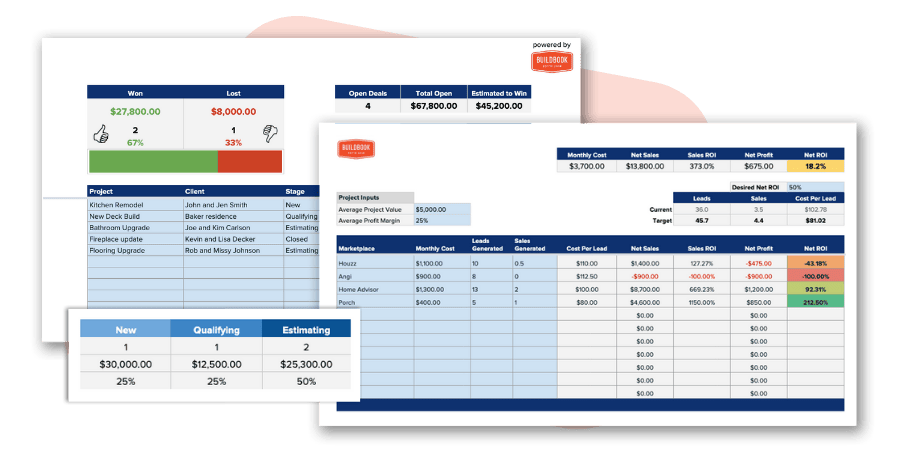# Profit Margin CalculatorDiscover what to charge and find your profit margin based on your markup percentage

## Want an even better profit margin calculator with more construction revenue tools?

This collection of construction calculators is 100% free to download, copy, and use as your own. You're free to modify and/or adjust any calculator to best fit the needs of you or your business.## What is profit margin?

In simple terms, a profit margin is the percentage of total amount charged that you will receive after overhead and constructions costs are paid. In other words, if you charge \$11,000 for a project which has \$9,000 in overhead and constructions costs to complete, your profit is what's remaining. (\$11,000 - \$9,000 = \$2,000). To find the profit margin of the project, you'd simply divide your profit by the total. (\$2,000/\$11,000 = .1818) Now, just multiply the result by 100 (.1818 x 100) and you'll see that your profit margin is 18.18%.

## What's the difference between profit margin and markup?

While profit is the amount of profit remaining after all overhead and construction costs are paid in a project, markup is the amount you increase to any overhead or construction costs. For example, if your construction costs are \$5,000, but you charge the client \$5,500, your markup would be \$500, or 10% (\$500/\$5,000 = .10). However, while your markup is 10%, your profit margin is only 9.09%. This is because your profit margin is based on the total amount you charge, not the total of amount of your expenses.

## What is the standard profit margin in a construction project?

While there are no rules on how much profit you can make on any given project, it seems like most residential home builders and remodelers agree that a 20% profit margin is standard. There are many factors such as the economy, type of project, unique client requirements, and much, much more that can drastically increase or decrease a projects profit margin, so it's best to consider all factors before setting your profit margin for any given project.